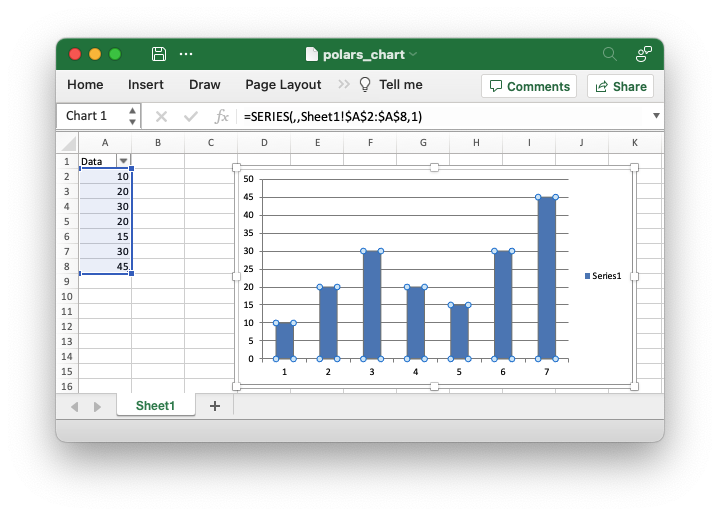# Example: Polars Excel output with a chart

An example of adding a Polars dataframe to a worksheet created by XlsxWriter and then adding a chart of the data. See Working with Polars and XlsxWriter for more details.```##############################################################################
#
# An example of adding a Polars dataframe to a worksheet created by XlsxWriter
# and then adding a chart of the data.
#
# Copyright 2013-2023, John McNamara, jmcnamara@cpan.org
#

import xlsxwriter
import polars as pl

df = pl.DataFrame({"Data": [10, 20, 30, 20, 15, 30, 45]})

with xlsxwriter.Workbook("polars_chart.xlsx") as workbook:
# Create the worksheet so we can reuse it later.

# Write the Polars data to the worksheet created above.
df.write_excel(workbook=workbook, worksheet="Sheet1")

# Create a chart object.